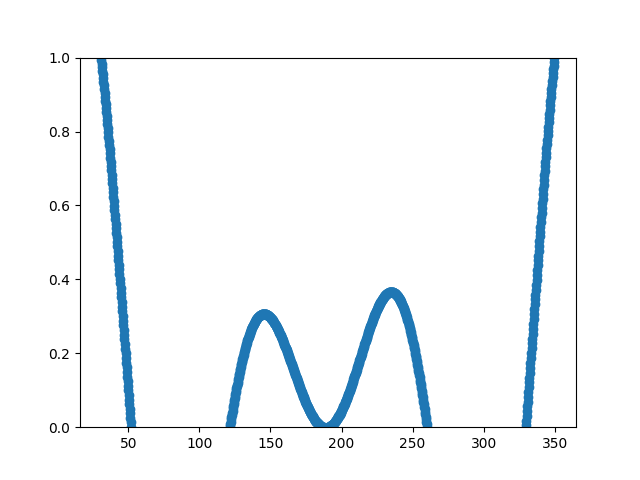# Resampling Data#

Downsampling lowers the sample rate or sample size of a signal. In this tutorial, the signal is downsampled when the plot is adjusted through dragging and zooming.

Note

This example exercises the interactive capabilities of Matplotlib, and this will not appear in the static documentation. Please run this code on your machine to see the interactivity.

You can copy and paste individual parts, or download the entire example using the link at the bottom of the page.import numpy as np
import matplotlib.pyplot as plt

# A class that will downsample the data and recompute when zoomed.
def __init__(self, xdata, ydata):
self.origYData = ydata
self.origXData = xdata
self.max_points = 50
self.delta = xdata[-1] - xdata

def downsample(self, xstart, xend):
# get the points in the view range
mask = (self.origXData > xstart) & (self.origXData < xend)
# dilate the mask by one to catch the points just outside
# of the view range to not truncate the line
# sort out how many points to drop
ratio = max(np.sum(mask) // self.max_points, 1)

# downsample data
xdata = xdata[::ratio]
ydata = ydata[::ratio]

print(f"using {len(ydata)} of {np.sum(mask)} visible points")

return xdata, ydata

def update(self, ax):
# Update the line
lims = ax.viewLim
if abs(lims.width - self.delta) > 1e-8:
self.delta = lims.width
xstart, xend = lims.intervalx
self.line.set_data(*self.downsample(xstart, xend))
ax.figure.canvas.draw_idle()

# Create a signal
xdata = np.linspace(16, 365, (365-16)*4)
ydata = np.sin(2*np.pi*xdata/153) + np.cos(2*np.pi*xdata/127)

fig, ax = plt.subplots()

# Hook up the line
d.line, = ax.plot(xdata, ydata, 'o-')
ax.set_autoscale_on(False)  # Otherwise, infinite loop

# Connect for changing the view limits
ax.callbacks.connect('xlim_changed', d.update)
ax.set_xlim(16, 365)
plt.show()


Gallery generated by Sphinx-Gallery